# Analytic continuation into a domain of a function given on part of the boundary

(diff) ← Older revision | Latest revision (diff) | Newer revision → (diff)

The following classical assertion is well known. Letbe a simply connected bounded domain with smooth boundary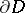, and. Then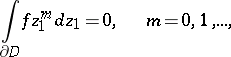(a1)

if and only if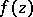extends into the domainas a holomorphic function of the class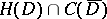. For the multi-dimensional case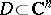, instead of the form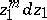, one takes an exterior differential form of class.

Ifis defined only on a part of the boundary of, then the existence of an analytic continuation intocannot be decided by the vanishing of some family of continuous linear functionals as in (a1). Solutions to this problem were given from the 1950s onwards by many mathematicians, see, e.g. [a1], [a2].

Some very simple solutions are given below.

1). Letbe the domain bounded by a part of the unit circle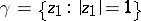and a smooth open arcconnecting two points ofand lying inside. Let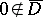. Set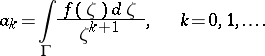Then the following assertion holds: If, then there is a function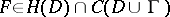such that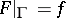if and only if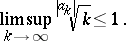(a2)

If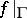is not identically zero, then (a2) is equivalent to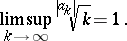2). Let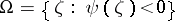be a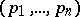-circular convex domain in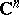, where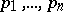are natural numbers, i.e.,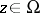implies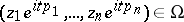for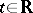. In particular, for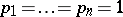this circular domain is a Cartan domain. Moreover, assume thatis convex and bounded and. Furthermore, letbe the domain bounded by a part ofand a hyper-surface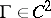dividinginto two parts and assume that the complement of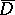contains the origin. Consider the Cauchy–Fantappié differential form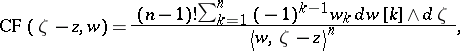where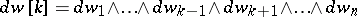,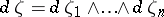,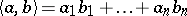. Then. By the Sard theorem,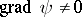for almost allon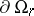, where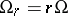is the homothetic transform of. Assume thatonand set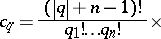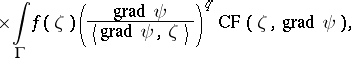where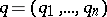,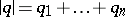,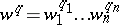. Let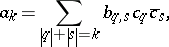where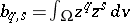and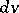is the volume element in. Here, all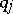and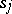are non-negative integers. Note that the integral moments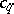depend onand, but the momentsdepend only on.

The following assertion now holds: For a function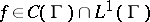to have an analytic continuationwith, it is necessary and sufficient that the following two conditions are fulfilled:

i)is a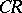-function on;

ii)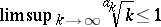.

A consequence of this is as follows. Letbe a bounded convex-circular domain (a Reinhardt domain). Set. For a functionto have an analytic continuation inas above it is necessary and sufficient that:

a)is a-function on;

b)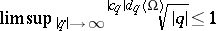.

How to Cite This Entry:
Analytic continuation into a domain of a function given on part of the boundary. Encyclopedia of Mathematics. URL: http://encyclopediaofmath.org/index.php?title=Analytic_continuation_into_a_domain_of_a_function_given_on_part_of_the_boundary&oldid=15858
This article was adapted from an original article by L. Aizenberg (originator), which appeared in Encyclopedia of Mathematics - ISBN 1402006098. See original article